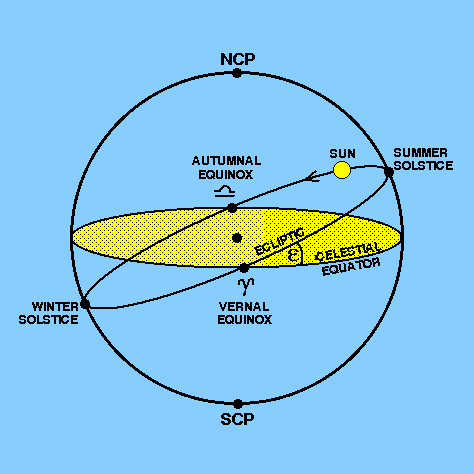The celestial sphere is an imaginary sphere of infinite radius centred on the Earth, on which all celestial bodies are assumed to be projected. This Earth-centred Universe is, of course, not an accurate model of the real Universe, so why introduce it? There are two reasons why the concept of a celestial sphere is useful. First, it forms a convenient pictorial representation of the different directions of astronomical objects, and second, calculations involving these directions can be performed using the formulae of spherical trigonometry. The celestial sphere is illustrated in Figure 9.

 figure 9: The celestial sphere (©Sky Publishing Corp.).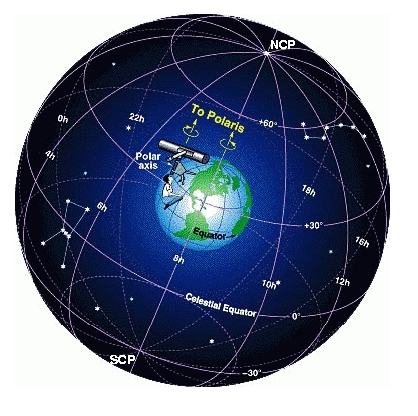The celestial sphere is assumed to be fixed, so as the Earth rotates the celestial sphere appears to rotate in the opposite direction once per day. This apparent rotation of the celestial sphere presents us with an obvious means of defining a coordinate system for the surface of the celestial sphere - the extensions of the north pole (NP) and south pole (SP) of the Earth intersect with the north celestial pole (NCP) and the south celestial pole (SCP), respectively, and the projection of the Earth's equator on the celestial sphere defines the celestial equator (CE). The celestial sphere can then be divided up into a grid in a similar manner to the way in which the Earth is divided up into a grid of latitude and longitude, as shown in Figure 9.

We can never observe the whole celestial sphere from the Earth as the horizon limits our view of it. In fact, we can only ever observe half of the celestial sphere at any one time, the half we observe depending on our position on the Earth's surface, as shown in Figure 10.

 figure 10: A northern-hemisphere observer's view of the celestial sphere.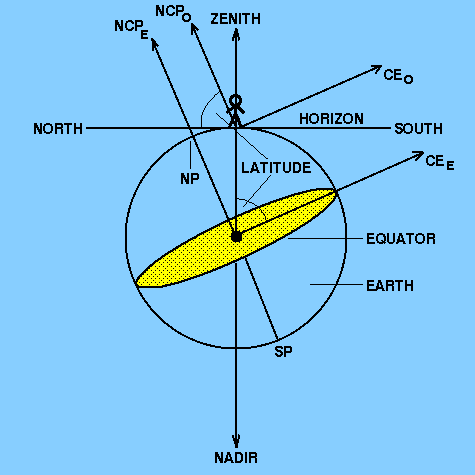In Figure 10, the observer has the impression of being on a flat plane and at the centre of a vast hemisphere across which the celestial bodies move. On all sides, the plane stretches out to meet the base of this celestial hemisphere at the horizon. The point directly overhead the observer (as defined by a line passing through the centre of the Earth and perpendicular to the horizon) is known as the zenith. The point opposite this, which the observer cannot see, is known as the nadir. Because the radius of the celestial hemisphere is infinite compared with the radius of the Earth, the directions of the north celestial pole and the celestial equator as viewed by the observer (NCPO and CEO) are indistinguishable from their real directions (NCPE and CEE), which are defined relative to the centre of the Earth. As the observer moves further north in latitude, the north celestial pole moves closer to the zenith until they become coincident when the observer is at the north pole. At the north pole, the celestial equator lies on the horizon. As the observer moves further south in latitude, the north celestial pole moves further away from the zenith until it lies at the horizon when the observer is at the Earth's equator. At the Earth's equator, the celestial equator passes through the zenith.

The stars are sufficiently distant that for our purposes we can assume that they are fixed to the celestial sphere. This is not true of solar system objects (e.g. the Sun, Moon, planets, comets, asteroids and spacecraft) which move (albeit slowly) with respect to the celestial sphere. We will return to the motion of solar system objects (and, in particular, the Sun) on the celestial sphere later. For now, we will concentrate on the motions of the stars.

The Earth rotates from west to east and hence the stars appear to revolve from east to west about the celestial poles on circular paths parallel to the celestial equator once per day. Some stars never set and remain visible at night all year. These are called circumpolar stars. A circumpolar star at its maximum altitude above the horizon is said to be at its upper culmination. Similarly, a circumpolar star at its minimum altitude above the horizon is said to be at its lower culmination. Stars further from the pole rise, attain a maximum altitude above the horizon (when they are said to transit) and then set below the horizon. These stars are visible at night only during that part of the year when the Sun is in the opposite part of the sky. Which stars are circumpolar depends on the latitude of the observer; stars within an angleof the north pole are circumpolar for an observer at northern-hemisphere latitude, and stars within an angleof the south pole are never seen by such an observer; the reverse is true for an observer in the southern hemisphere. This means that for observers at the Earth's poles, all of the stars are circumpolar and the observers never see any of the stars in the opposite hemisphere. For observers at the Earth's equator, none of the stars are circumpolar and the observers see the whole celestial sphere during the course of a year. A circular gap in the ancient constellation figures, centred on the south pole, shows that the people who named the constellations must have lived at a latitude of about 36°, probably in the eastern Mediterranean. The above concepts are illustrated in Figure 11.

 figure 11: The motion of the stars as viewed by a northern-hemisphere observer.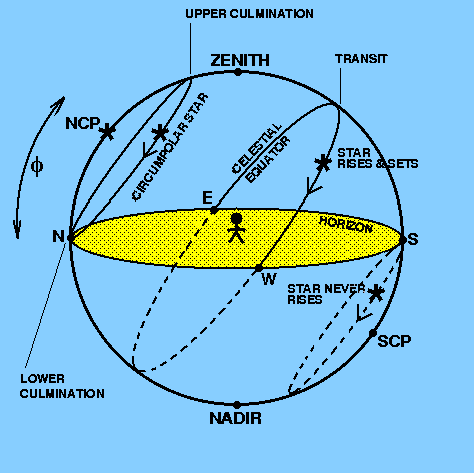As mentioned above, the Sun not only revolves with the stars on the celestial sphere each day, it also moves much more slowly along a path relative to the stars, making one revolution of this path in one year. This motion is due to the fact that the Earth makes one rotation of the Sun each year and because the Sun is much closer to the Earth than the stars. An observer who notes each month what group of stars is first visible above the western horizon after sunset will notice that there is a regular progression along a strip in the celestial sphere. The constellations along this strip are known as the signs of the zodiac.

The apparent path of the Sun in the sky is known as the ecliptic and is actually the intersection of the plane of the Earth's orbit with the celestial sphere. Because the rotation axis of the Earth (which defines the celestial sphere) is tilted at an anglewith respect to the plane of the Earth's orbit, the ecliptic is inclined at an angleto the celestial equator, as shown in Figure 12. The angleis known as the obliquity of the ecliptic and is currently about 23°27´.

The ecliptic and the equator intercept at two points, associated with the zodiacal constellations of Aries and Libra. The first point of Aries,, is defined to be the point where the Sun, moving along the ecliptic, crosses the celestial equator from south to north. This occurs at the spring equinox (or vernal equinox), around March 20, when day and night are of approximately equal length (hence the name), although strictly speaking it is when the same latitude north and south of the equator experiences days of equal length. The first point of Aries is sometimes referred to as the vernal (or spring) equinox and marks the beginning of spring in the northern hemisphere. Day and night are also of approximately equal length at the autumnal equinox, around September 22, when the Sun crosses the celestial equator from north to south in the constellation of Libra,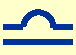. The autumnal equinox marks the beginning of autumn in the northern hemisphere.

The maximum altitude of the Sun in the sky, as viewed from the northern hemisphere, gradually increases from the spring equinox until it reaches a maximum around June 21 - the summer solstice (when the Sun appears to `stand still' in the sky before starting to move back towards the celestial equator). At the summer and winter solstices the Sun is directly overhead at noon at the Tropics of Cancer and Capricorn, respectively, these being the zodiacal constellations associated with those parts of the ecliptic where the Sun is at these times. The summer solstice marks the beginning of northern hemisphere summer. Similarly, the Sun reaches its minimum altitude in the sky when viewed from the northern hemisphere around December 21 - the winter solstice - which marks the beginning of northern hemisphere winter. These concepts are illustrated in Figure 12. It should be noted that the date that the equinoxes and solstices fall on in each year varies slightly due to, amongst other things, the insertion of leap days. For example, in 2007, the equinoxes fell on March 21 and September 23, and the solstices fell on June 21 and December 22.

The first point of Aries forms the basis of the most often used celestial coordinate system, which is where we shall turn to now.

 figure 12: The ecliptic.